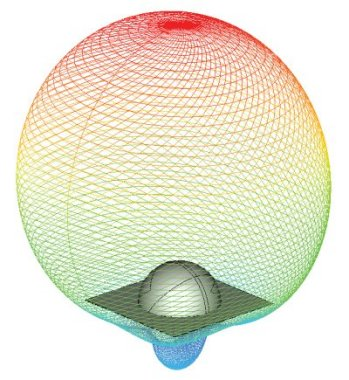# Antenna Radiation Characteristics## Antenna Radiation Characteristics:

CHRONOS and SINGULA successfully compute the radiation characteristics of antennas of different types: linear antennas, microstrip antennas, dielectric resonator antennas, horn antennas, reflector antennas and others.

CHRONOS can calculate the radiation characteristics at many frequencies within the frequency band of the input transient pulse from a single run.

Our software can accurately calculate far zone radiation patterns of different components of electric and magnetic fields in the form of a 2D polar plot, a 2D rectangular plot or a full 3D plot. SINGULA calculates precisely the phase centre, input impedance and radiation efficiency of an antenna. The program also determines the power loss in a dielectric volume or the ohmic loss in a conducting volume.

CHRONOS and SINGULA analyze the effect of the presence of thin dielectric surfaces and/or thick dielectric volumes, arbitrarily shaped objects of finite and/or infinite conductivities on the radiation characteristics. Polarization characteristics such as axial ratio and tilt angle plots as a function of observation angle can be easily obtained.